# 9+ Sample Basic Metric Conversion Chart Templates

The metric system has been around for ages and has been devised in such a way to make our day to day calculations much simpler. A basic Metric Conversion Chart Templates allows you to carry out simple conversions between units of length, height, distance or weight. All you have to do is insert your amount and the desired unit you want the amount in. So if maths isn’t exactly your strong point, these charts can come to your rescue.

## Sample Basic Metric System Conversion Chart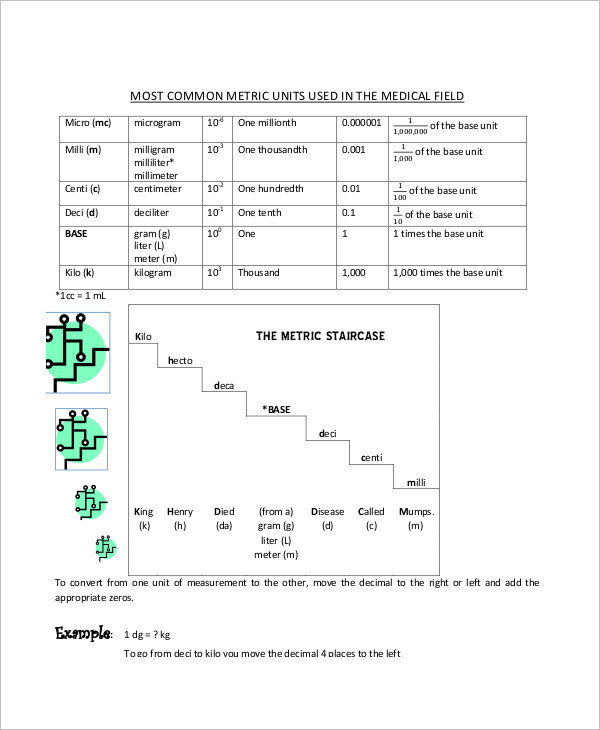laniertech.edu

A basic metric system conversion Chart Templates comes with the bare minimums that are required. It can be used to carry out any simple calculations in this arena in a neat and organized fashion.

## Basic Metric Unit Conversion Chart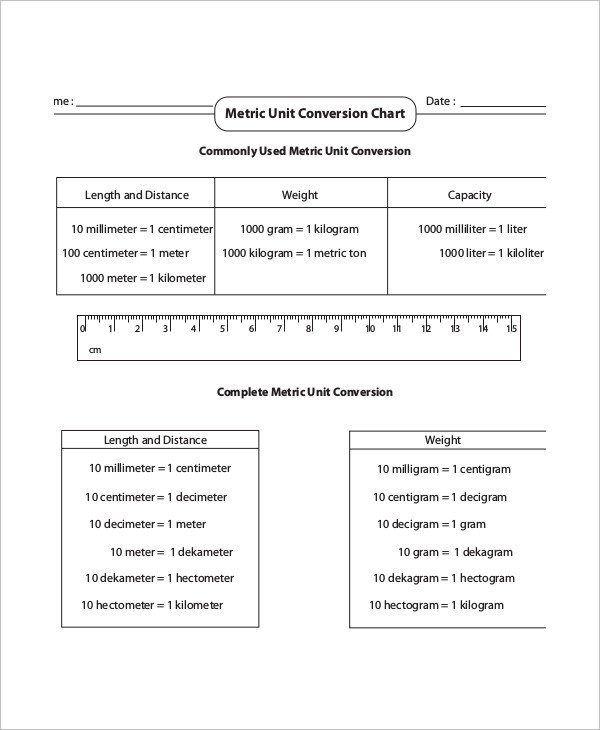mathworksheets4kids.com

The basic metric unit conversion chart allows you to convert between the various groups of units like the International System of Units and the commonly used ones. Such basic calculations can be carried out with ease.

## Basic Metric Measurement Conversion Chart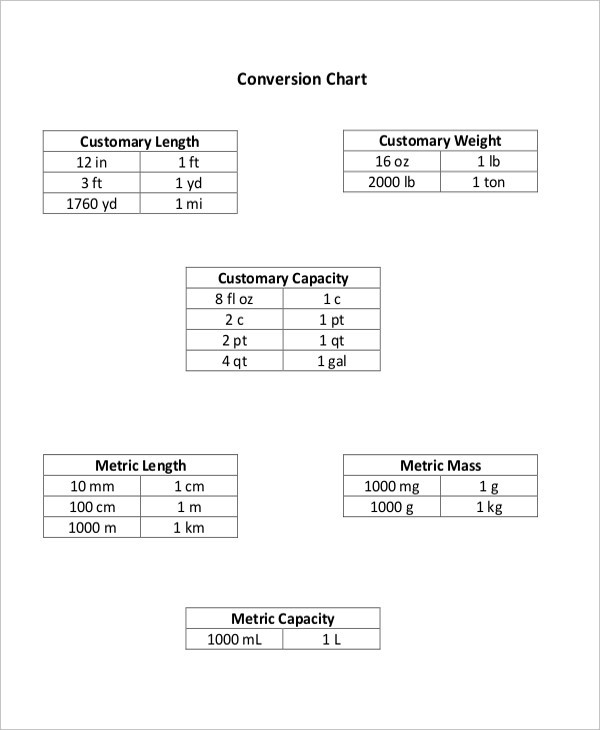wccusd.net

If you have to carry out any conversions or mathematical calculations related to the field of measurements and metric units, you can do so with this basic metric measurement conversion chart.

## Metric Base Conversion Chart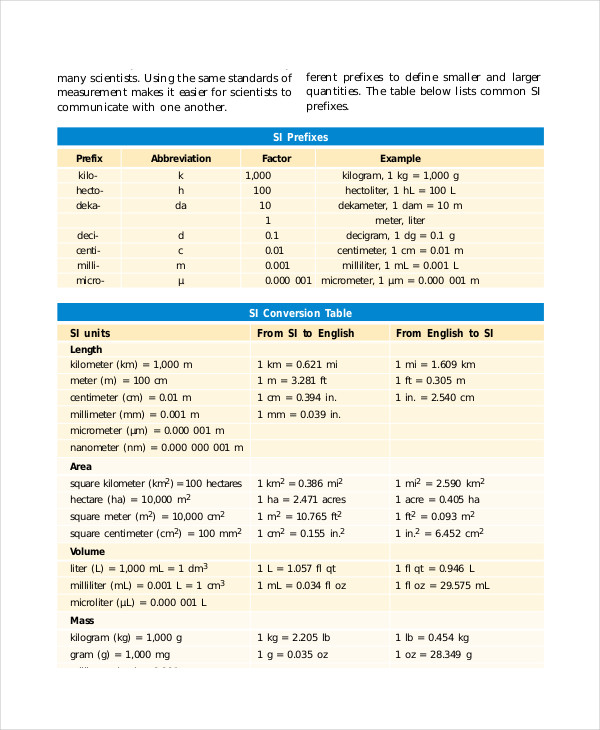go.hrw.com

This metric base conversion chart is multipurpose and would help you carry out complicated calculations in no time. Also, the metric base conversion chart allows you to proceed with the conversion in an organized manner.

## Basic Metric Length Conversion Chart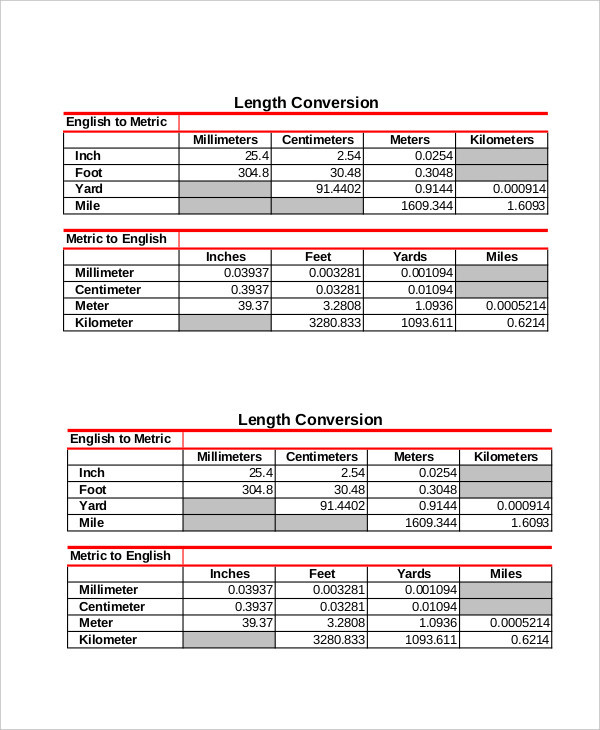labtesting.com

## Basic Math Metric Conversion Chart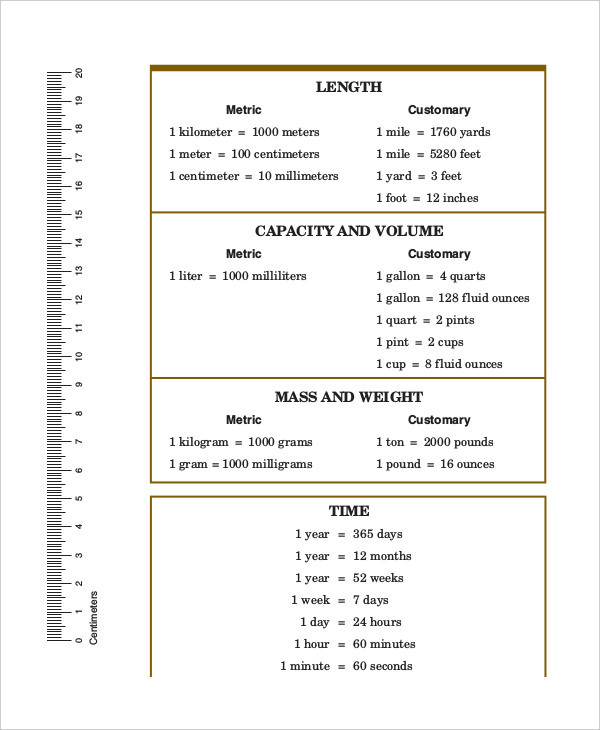tarleton.edu

## Basic Metric Imperial Conversion Chart Template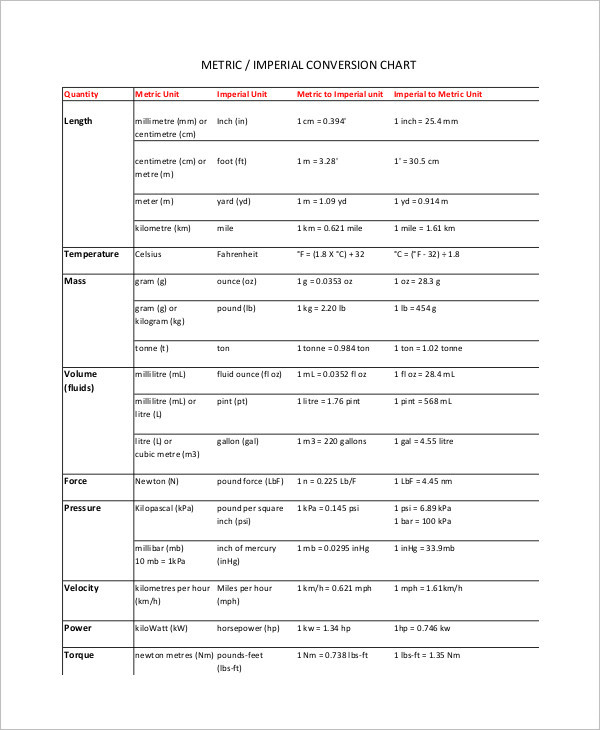afiaustralia.com.au

## Basic Metric Conversion Chart Example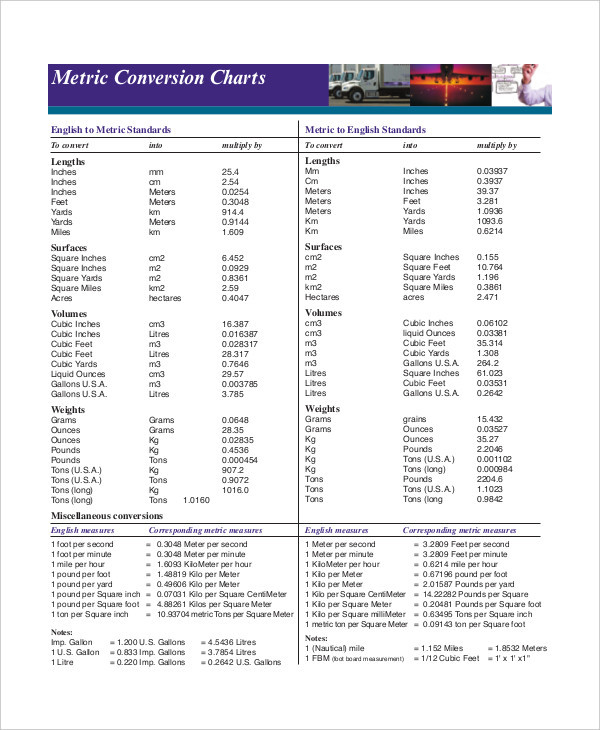stevensglobal.com

## Basic Metric Conversion Data Chart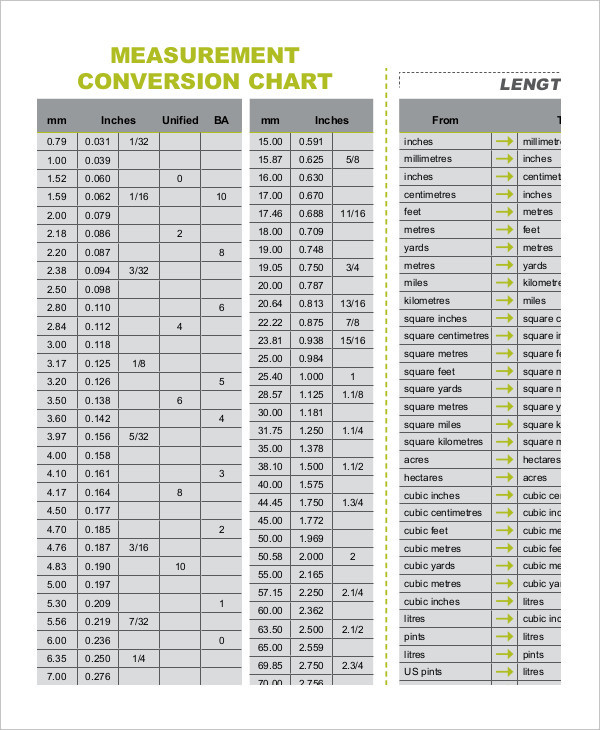meredithdist.com.au

## Base Metric to English Conversion Chart

This base metric to English conversion chart may be used to make tough calculations and to carry out complicated conversions. It can be used in a versatile manner as well.

### > How can you use the Basic Metric Conversion Chart?

The basic conversion charts are rather simple to use. For example, let’s say you have to convert your weight from kilogram to gram or you have to convert your height from centimeters to inches. Carrying out such complicated conversions can be quite tricky if you are doing them in your head. Instead, you can take the help of these conversion charts.

Here, the format has been provided to you already. You just have to insert the numbers in the rightful places. You can use the basic metric conversion charts to carry out different kinds of mathematical calculations in a neat and well chalked out manner. You can also see Metric System Conversion Chart Templates.

### > What are the benefits of the Basic Conversion Charts?

The basic conversion charts here come with a wide range of benefits. Some of them are:

• If you have impending calculations which are tricky and time-consuming, t these charts can make your work easier for you. They would significantly reduce your hassle and would also save you time and energy.
• Any prolonged calculations can be carried out in these charts. All you have to do is insert the numbers and the desired units you want them in.
• The basic conversion charts can be used by anyone for a number of purposes. They are pretty flexible and versatile that way. You can also see Simple Metric Conversion Chart Templates.

If you have been looking for a way to convert between units without too much of a hassle, then basic metric conversion charts are a must. In case you have to convert between the SI system and a bunch of units that aren’t commonly used, then you should use the charts to make sure your calculations are spot on.